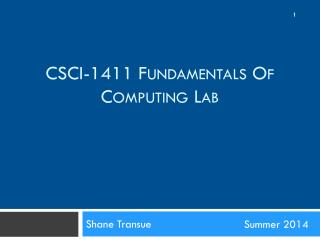DownloadDownload PresentationShane Transue

# Shane Transue

Download Presentation## Shane Transue

- - - - - - - - - - - - - - - - - - - - - - - - - - - E N D - - - - - - - - - - - - - - - - - - - - - - - - - - -
##### Presentation Transcript

1. CSCI-1411 Fundamentals of Computing Lab Shane Transue Summer2014

2. Lab 2: Introduction to the C++ Programming Language • Overview: • Lab 2 Components • Lab Sections (2.1, 2.2, 2.3, 2.4) • Lab 2 Concepts • Printing • Constants • Arithmetic (C++ data-types, operators)’ • int – Integer Types • float– Floating point, Double (decimal representations) • Characters and Strings • char– Represents a single character • string – Represents a sequence of characters (textual information) • Not a built in ‘type’

3. Lab 2: Introduction to the C++ Programming Language • C++ Constants • Defines a constant that will not change during execution • Ex: e, pi, etc • Removes ‘magic numbers’ from code: int value = 4 * 5; // What is value? What does 4 represent? 5? const intRECTANLGE_WIDTH = 4; // For some reason the rectangle const int RECTANGLE_HEIGHT = 5; // dimensions never change. int area = RECTANGLE_WIDTH * RECTANLGE_HEIGHT; // Rectangle Area • Makes code explicit and easier to read • Again, it’s for humans, the computer doesn’t care

4. Lab 2: Introduction to the C++ Programming Language • C++ Arithmetic • Similar to mathematical notation • You would generally write: x = 4, and alternatively: 4 = x • Only one of these is valid in C++ • int value = 4; // Valid • 4 = int value; // Invalid (Maybe in English the equivalent would be something like “Four is an integer value”, but to C++ this is invalid syntax • Ensure to properly space your equations: • int value = 44*4-3+3(3+4/4)-2*(3); • int value = 44 * (4 – 3) + 3 * ((3 + 4) / 4) – 2 * 3; • Why does this still have a problem? • Compiler is always the ultimate test (for syntax)

5. Lab 2: Introduction to the C++ Programming Language • C++ Characters and Strings • Characters are a ‘built-in’ primitive type char Allocates at a minimum 1 byte of memory (per C++ standard) • Contains ASCII equivalent when converted to an integer • Chars are defined with single quotes: charmyChar = ‘A’; intcharAsASCII = myChar; // Valid • Printing charAsASCII to the terminal will result in the following: cout << charAsASCII << endl; • 65 (Is printed to the terminal)

6. Lab 2: Introduction to the C++ Programming Language • C++ Strings • Not a ‘built-in’ primitive • Built-in C++ standard class • Abstraction of dealing with sequences of characters • Text, Sentences, etc. • Defined with double quotes: string myText = “HelloWorld”; • Easily printed to the terminal using the cout << operator: cout << myText << endl; • Will display: Hello World in the terminal

7. Lab 2: Introduction to the C++ Programming Language • 2.1 Working with the coutStatement • (name.cpp) • 2.2 Working with Constants, Variables, and Arithmetic Operators • (circlearea.cpp) • 2.3 Rectangle Area and Perimeter • Don’t forget to create the source file: • (rectangle.cpp) • 2.4 Working with Characters and Strings • (stringchar.cpp)

8. Lab 2: Introduction to the C++ Programming Language • Submission File Checklist • Submit all files on Canvas (One at a time or all of them in a single zip file). Be sure to include all source files and documents. • 2.1 name.cpp • 2.2 circlearea.cpp • 2.3 rectangle.cpp • 2.4 stringchar.cpp • Don’t forget to answer any questions from the exercises in a comment block at the end of your code